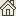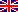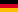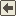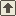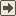## CENCO 71266 tube for the measurement of the charge-to-mass ratio e/m of the electron Tube pour la détermination de la charge spécifique e/m de l'électron Röhre für die Bestimmung von e/m des Elektrons

CENCO (Central Scientific Company) was bought by Sargent-Welch, a supplier of science education equipment and materials.

The CENCO e/m tube is a triode in which electrons are emitted by an indirectly heated cathode and accelerated by means of a potential difference between cathode and plate. An electron beam is formed when electrons pass through a small hole in the center of the plate into the experimental region of the tube. Since the beam of electrons would normally diverge, an additional grid electrode is placed between cathode and plate to change the shape of the accelerating field so as to produce a parallel or converging electron beam.

A uniform magnetic field is produced by a pair of circular Helmholtz coils.

The bulb and disk are coated with a material which fluoresces when struck by electrons. The tube contains traces of argon and mercury that aid in focusing the electron beam as well as to cause the beam to make a visible trace.

When a particle moves along a circular path, it is constanly accelerating, and there must be a centripetal force producing this acceleration. This force is given by the following equation: F= mv²/r, where m is the particle's mass, v is its speed, and r is the radius of its circular path. By applying a magnetic field of strength B perpendicular to the velocity of the moving electrons, a centripetal force of strength F = evB is produced, Where e is the charge of a single electron. By combining the two equations above, we get the following expression for the charge over mass ratio of the electron: e/m = v/Br.

Fiche technique • Data sheet • Datenblatt: CENCO_71267.pdf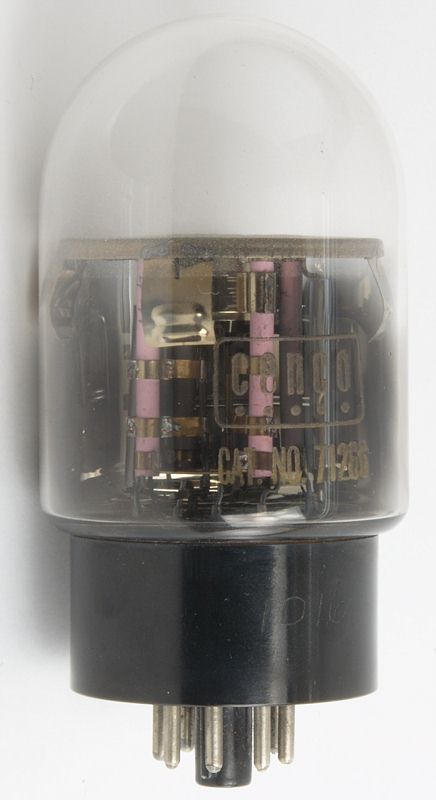Hauteur • Height • Höhe : 10,6 cm • 4" 3/16
Diamètre • Diameter • Durchmesser : 5,5 cm • 2" 3/16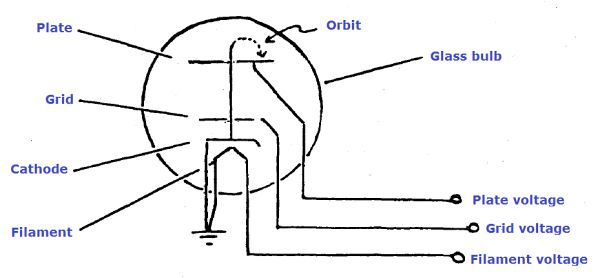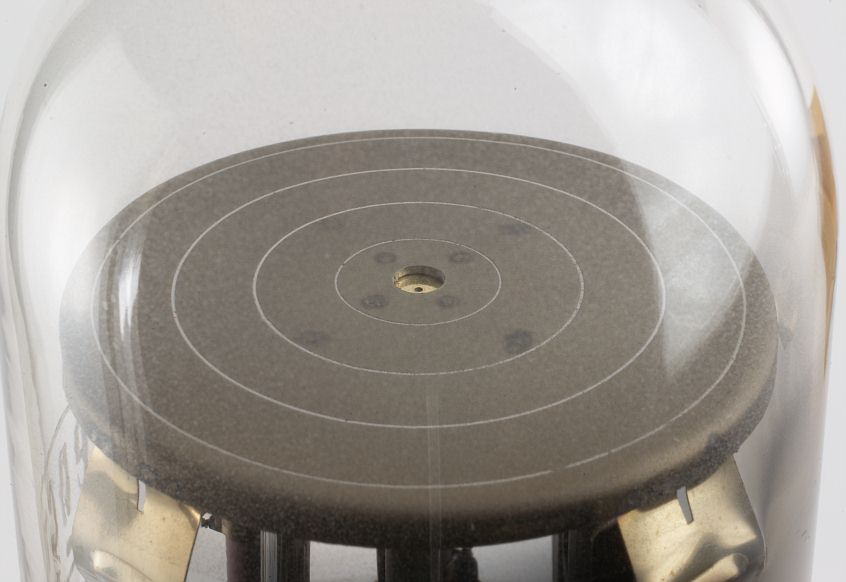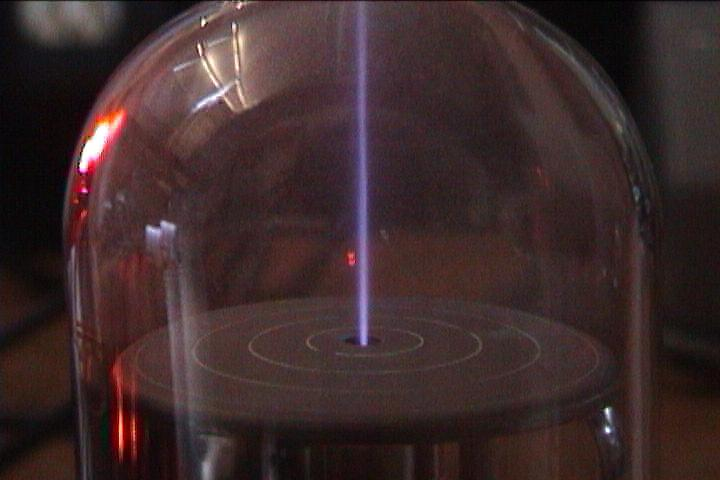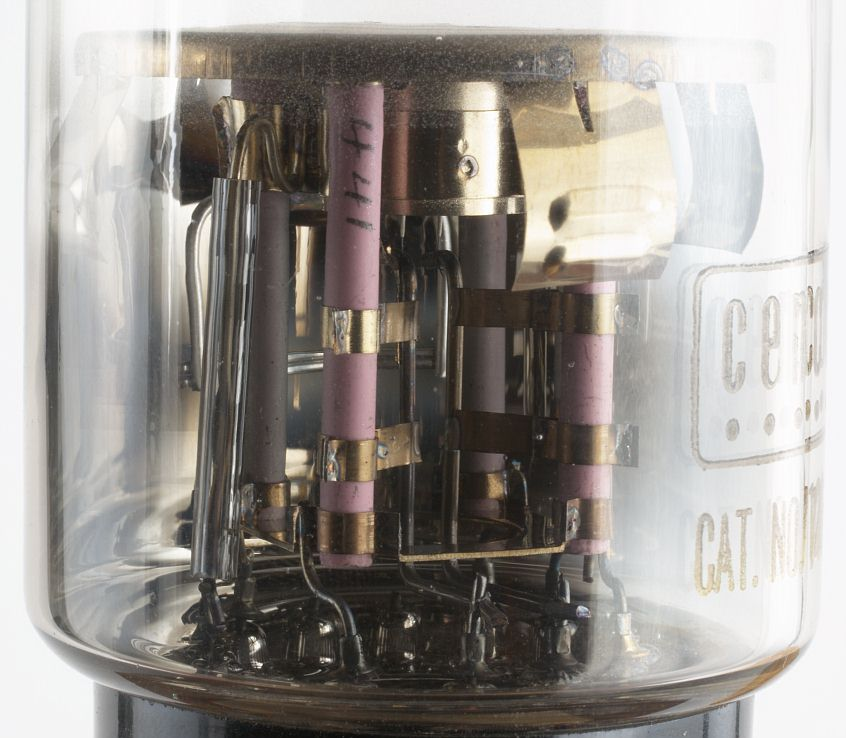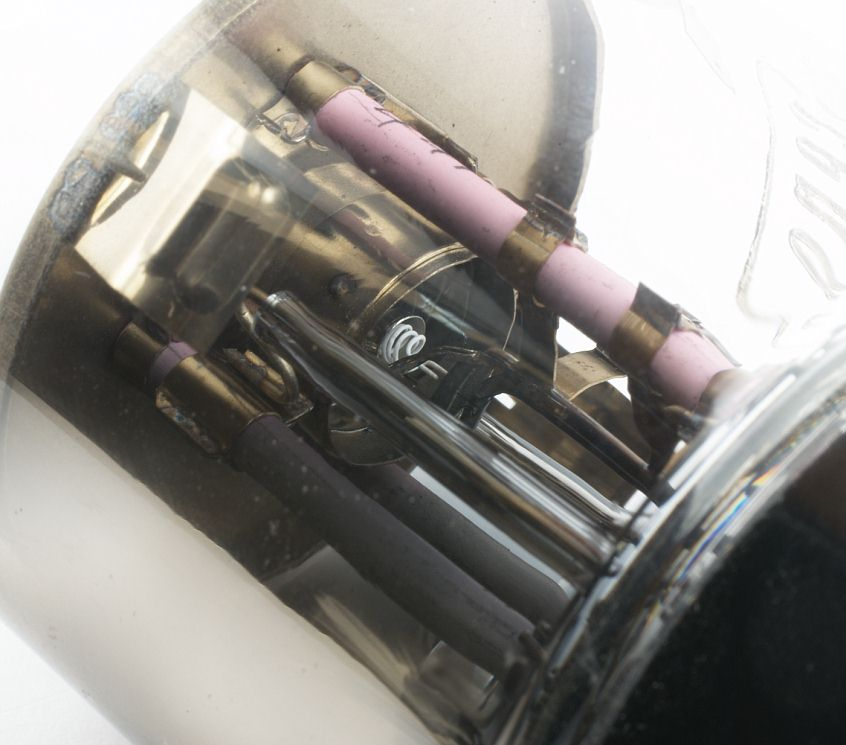Le contenu de ce site est sous copyleftThe content of this site is under copyleftDer Inhalt dieser Website steht unter Copyleft